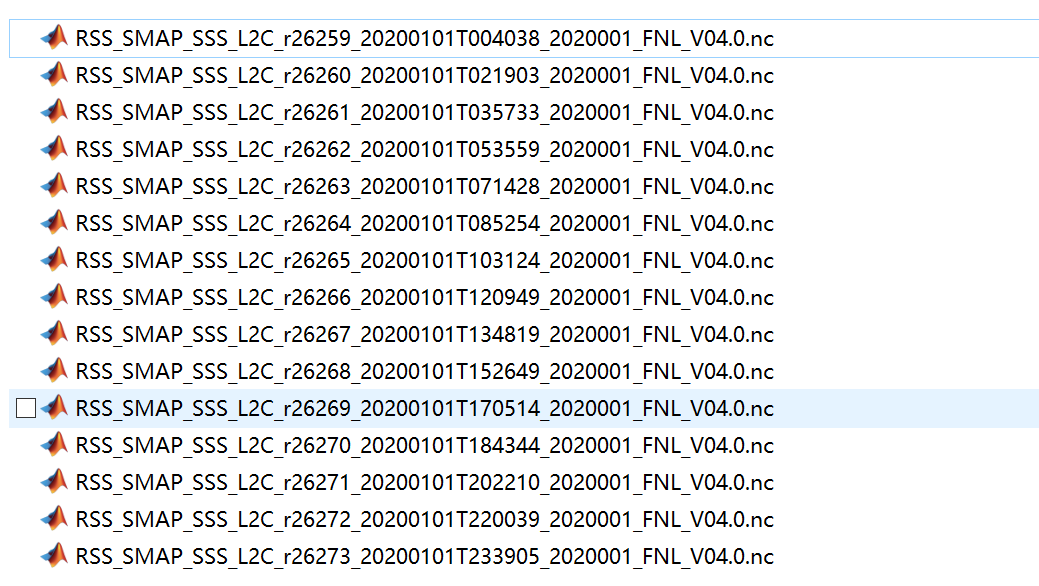### python 批量处理nc文件-字符串拼接文件 ，有规律文件名##拼接文件名

``````str1='RSS_SMAP_SSS_L2C_r'
str2='*'#r26259_
str3='_FNL_V04.0.nc'
str4='*'
``````

``````import calendar
``````

``````for year in range(2020,2021):#这里只展示2020年

for month in range(1,13):#表示12个月

for day in range(1,calendar.monthrange(year,month)+1):#表示每个月的天数

``````

``````import glob
``````

``````path='G:\\'#文件路径，一般需要两个\，别问为什么	，不用可能报错
for year in range(2020,2021):

for month in range(1,2):
# for day in range(1,calendar.monthrange(year,month)+1):
for day in range(1,3):

#我的文件放在01号文件夹下面，所以拼接的时候加入了：'%02.0f'%month+'\\'
fn='%02.0f'%month+'\\'+str1+str2+'_'+'%04.0f'%year+'%02.0f'%month+'%02.0f'%day+\
'T'+str4+'_'+str4+str3
f=glob.glob(path+fn)  #这里需要绝对路径

``````

``````for file in f:
f1 =nc.Dataset(file,'r')
# print(f1)
lat = `f1.variables['cellat'][:].data`
lon = f1.variables['cellon'][:].data
time = f1.variables['time'][:].data
``````

``````import calendar
import glob
import netCDF4 as nc

str2='*'
str3='_FNL_V04.0.nc'
str4='*'
path='G:\\'#文件路径
for year in range(2020,2021):

for month in range(1,13):
for day in range(1,calendar.monthrange(year,month)+1):
fn='%02.0f'%month+'\\'+str1+str2+'_'+'%04.0f'%year+'%02.0f'%month+'%02.0f'%day+\
'T'+str4+'_'+str4+str3
f=glob.glob(path+fn)

for file in f:
f1 =nc.Dataset(file,'r')
# print(f1)
lat = f1.variables['cellat'][:].data
lon = f1.variables['cellon'][:].data

``````
``````                                一个努力学习python的海洋小白
水平有限	，欢迎指正！！！
欢迎评论	、收藏。
``````